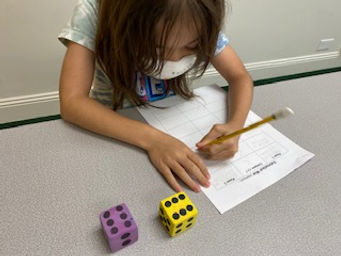## Ms. Dawn

### Target 1​

###### Lesson Type:

Continuation

Number Operation

:

Computation

Estimate a given quantity based on place-value understandings.

###### 1:

Estimate quantities and use one-to-one correspondence to check the accuracy of the estimate.

###### 2:

Understand the value of using a point of reference when estimating quantity.

###### 3:

Understand that rounding and estimation are a way to quickly check the accuracy of computations.

###### 4:

Understand that you can use estimation when an exact answer is not needed.

###### 5:

Develop a strategy for estimating quantities (i.e., developing a unit of measurement for estimating – if this is what 10 units looks like this must be close to 40 units).

3rd

###### Vocabulary:

Estimate, Quantity, Place Value, Round

Activities:

1. Students played a game called "Estimation War." They rolled 2 dice and created 2-digit numbers. They had to then use rounding as an estimation strategy. Then, compare their answer with their partner. Whoever had the higher estimate won the point. The students did it for addition, subraction, and multiplicaiton.

2. Students solved double digit multiplicaiton problems using estimation.

3. Students pretended to create a shopping list for Christmas. They needed to buy gifts for 4 people. They looked at ads online for stores and wrote the actual price it was on sale for and then estimate how much it would cost for the item. Once figuring out the estimate for all four people, students needed to estimate about how much money they would need for all their presents.### Home Exploration

###### Challenge Problem:

Given a grocery list of items, have students look up the price it would cost in a store ad for each item. Then, have them estimate and tell you about how much money they would need for all those items.

###### Guiding Questions:

What does it mean to estimate? How would I round those prices to make an estimate for each item?## Absent Students:

### Target 2

:

###### 1:

Identify and complete a growing pattern, where something new is added to each segment.

###### 2:

Identify a pattern two or more attributes are changed in each segment.

3rd

###### Vocabulary:

Activities:

1. Students needed to find the error in each pattern table .They needed to use their knowledge about number patterns and find what needed to changed to make it correct and the pattern flow.

2. Students rolled a die and used that number to create addition patterns when given a starting number. Then, create subtraction patterns when given a starting number.

3. Students rolled a die to insert numbers into two different boxes that had students multiply and then add to create patterns.### Home Exploration

Roll a die twice, create a pattern starting with a given number. The first box will be addition and second box subtraction. You may change up the operations.

What is a pattern? What is the rule? How do you know?

###### Guiding Questions:### Target 3

:

###### Vocabulary:

Activities:### Home Exploration